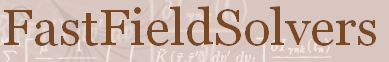FastFieldSolvers Forum Home | Profile | Register | Active Topics | Members | Search | FAQ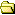All Forums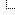FastFieldSolvers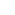FastModel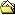FastModel Ignores .Units

Note: You must be registered in order to post a reply.

Screensize:
Antispam question: What do MOONwalk and MOONdance have in common?
Format Mode: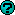Format: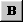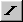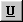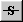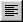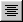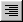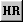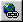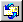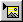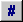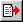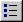T O P I C    R E V I E W BillBucket Posted - Oct 03 2017 : 01:57:58 I'm modeling two coils using input data with two different units. This isn't a problem for FastHenry as the .Units command is interpreted correctly.I can see how FastModel wouldn't normally need units, since none are shown nor need to be to get an idea of relative sizes. This seems to fail when using two different units in a single FH input file. Is this expected behavior? Is the only work around to convert the units to being the same?Thanks.Here's an example file that shows the problem for FastModel:``` *Unitless Model* .default nwinc=1 nhinc=1 h=10 w=10 .default z=0 .Units um N111 x=100 y=0 z=100 N112 x=100 y=100 z=100 E11 N111 N112 N121 x=100 y=100 z=100 N122 x=0 y=100 z=100 E12 N121 N122 N131 x=0 y=100 z=100 N132 x=0 y=0 z=100 E13 N131 N132 N141 x=0 y=0 z=100 N142 x=90 y=0 z=100 E14 N141 N142 .external N142 N111 .Units mm N211 x=100 y=0 z=0 N212 x=100 y=100 z=0 E21 N211 N212 N221 x=100 y=100 z=0 N222 x=0 y=100 z=0 E22 N221 N222 N231 x=0 y=100 z=0 N232 x=0 y=0 z=0 E23 N231 N232 N241 x=0 y=0 z=0 N242 x=90 y=0 z=0 E24 N241 N242 .external N242 N211 .freq fmin=1e6 fmax=1e6 .end``` 2   L A T E S T    R E P L I E S    (Newest First) BillBucket Posted - Oct 03 2017 : 18:12:27 Thanks Enrico. I'll take a look at the source. In the mean time converting the affected units isn't too much trouble. Enrico Posted - Oct 03 2017 : 08:46:59 It is expected behavior as this feature is not at present supported by FastModel. However, as we provide the full source code of FastModel, you have the full control on the funcionality and you may, if you have some coding skill, implement the feature yourself.As an alternative, if you need it on a short schedule, we are fully willing to extend FadtModel based on your specifications as a consulting service. In this case, kindly contact us via any of the channels indicated in the "Contacts" page.Best Regards,Enrico
 FastFieldSolvers Forum © 2020 FastFieldSolvers S.R.L.